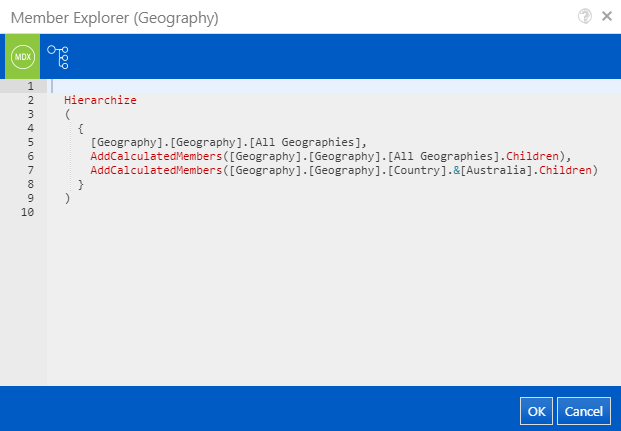# MDX Mode¶

For advanced usages of the Member Explorer, MDX mode shows the raw query that will be run and allows that query to be edited in order to give fine grained control to the user.

To view the MDX for the current members in the Member List, press the MDX button in the toolbar and the Member Explorer will change to show a pane with the MDX.The MDX pane is editable so that the MDX can be updated.

## Dynamic Subsets using MDX¶

One benefit of being able to edit the MDX is to allow the creation of dynamic subsets such as the last 14 days or last 3 months.

The following example uses a few techniques to get the last 14 days from the `[Date].[Calendar]` hierarchy:

• Use the MDX StrToMember function to construct two MemberUniqueID values from strings
• Inside the strings, the in-built Format function is used to format the current date/time into a format that the hierarchy uses as a key, i.e. `yyyyMMdd` becomes `20190323` and then that becomes the member `[Date].[Calendar].[Date].&`
• Then the MDX Lag function is used to make the first member the member 13 before now
• That is all joined together by the MDX Range `:` operator which means from this member to that memeber and all members in-between.
• Finally the members are ordered in descending order by the MDX Order function so that are ordered from the most recent to the least recent date based on their `Member_Key`.
``````Order
(
{
StrToMember('[Date].[Calendar].[Date].&[' + Format(Now(),'yyyyMMdd') + ']').Lag(13)
:
StrToMember('[Date].[Calendar].[Date].&[' + Format(Now(),'yyyyMMdd') + ']')
},
[Date].[Calendar].CurrentMember.Member_Key,
DESC
)
``````